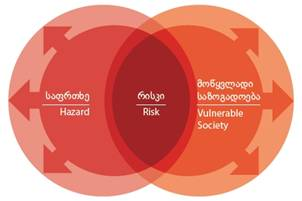Risk AssessmentThe risk assessment method is a semi-quantitative method that estimates the level of expected losses for a certain reference period, using the following equation:

Risk = H * V * A

H = Hazard, expressed as the chance that a basic unit of 1 hectare in one of the hazard classes is actually hit by a hazard event in a reference period of 50 years. This is referred to as the spatial probability of occurrence;

V = Physical vulnerability of the particular element-at-risk, expressing the probability of complete loss of the elements at risk of the same class given the occurrence of the hazard event.

A = Amount of exposed elements-at-risk, calculated by overlaying in a GIS the hazard classes with the elements at risk and calculating the number of elements at risk per hazard class in each community.

First we have calculated the amount of exposure of different elements-at-risk (buildings, population, GDP, forest, crops and protected areas) for each of the 10 hazard types (earthquakes, floods, landslides, mudflows, rockfall, snow avalanches, wildfires, drought, hailstorms and windstorms).  The exposure is calculated for the three classes of each of the hazard maps (High, moderate and low).

The hazard is expressed as the spatial probability that a certain area is expected to be impacted by one of the hazard types within a given reference period. In this study we used a reference period of 50 years. In order to estimate the spatial probability of occurrence, we analyzed the historical catalogues of the various hazard types.

Next we estimated the area affected by one event (e.g. for landslide an area of 5000 m2) and multiplied this with the number of expected events. The resulting value expresses the estimated area that might be impacted by a specific hazard in a reference period of 50 years. This was then divided by the total area of the hazard class in order to obtain the spatial probability of occurrence.

The vulnerability is evaluated as the degree of damage that is likely to occur to a particular element-at-risk given the impact of a certain type of hazard. Given the national scale and the fact that we cannot differentiate the degree of intensity we have adopted single vulnerability values for each of the elements-at-risk and hazard types.